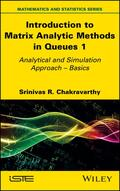John Wiley & Sons Introduction to Matrix Analytic Methods in Queues 1Matrix-analytic methods (MAM) were introduced by Professor Marcel Neuts and have been applied to a v.. Product #: 978-1-78630-732-3 Regular price: \$142.06 \$142.06 Auf Lager

# Introduction to Matrix Analytic Methods in Queues 1

## Analytical and Simulation Approach - Basics1. Auflage November 2022
368 Seiten, Hardcover
Wiley & Sons Ltd

ISBN: 978-1-78630-732-3
John Wiley & Sons

Jetzt kaufen

### Preis: 152,00 €

Preis inkl. MwSt, zzgl. Versand

Matrix-analytic methods (MAM) were introduced by Professor Marcel Neuts and have been applied to a variety of stochastic models since. In order to provide a clear and deep understanding of MAM while showing their power, this book presents MAM concepts and explains the results using a number of worked-out examples.

This book's approach will inform and kindle the interest of researchers attracted to this fertile field. To allow readers to practice and gain experience in the algorithmic and computational procedures of MAM, Introduction to Matrix Analytic Methods in Queues 1 provides a number of computational exercises. It also incorporates simulation as another tool for studying complex stochastic models, especially when the state space of the underlying stochastic models under analytic study grows exponentially.

The book's detailed approach will make it more accessible for readers interested in learning about MAM in stochastic models.

List of Notations ix

Preface xi

Chapter 1 Introduction 1

1.1. Probability concepts 2

1.1.1. Random variables 5

1.1.2. Discrete probability functions 6

1.1.3. Probability generating function 7

1.1.4. Continuous probability functions 7

1.1.5. Laplace transform and Laplace-Stieltjes transform 9

1.1.6. Measures of a random variable 10

1.2. Renewal process 11

1.2.1. Renewal function 12

1.2.2. Terminating renewal process 15

1.2.3. Poisson process 16

1.3. Matrix analysis 18

1.3.1. Basics 18

1.3.2. Eigenvalues and eigenvectors 23

1.3.3. Partitioned matrices 27

1.3.4. Matrix differentiation 28

1.3.5. Exponential matrix 30

1.3.6. Kronecker products and Kronecker sums 32

1.3.7. Vectorization (or direct sums) of matrices 33

Chapter 2 Markov Chains 35

2.1. Discrete-time Markov chains (DTMC) 36

2.1.1. Basic concepts, key definitions and results 36

2.1.2. Computation of the steady-state probability vector of DTMC 43

2.1.3. Absorbing DTMC 45

2.1.4. Taboo probabilities in DTMC 47

2.2. Continuous-time Markov chain (CTMC) 48

2.2.1. Basic concepts, key definitions and results 48

2.2.2. Computation of exponential matrix 52

2.2.3. Computation of the limiting probabilities of CTMC 57

2.2.4. Computation of the mean first passage times 58

2.3. Semi-Markov and Markov renewal processes 61

Chapter 3 Discrete Phase Type Distributions 71

3.1. Discrete phase type (DPH) distribution 72

3.2. DPH renewal processes 92

3.3. Exercises 97

Chapter 4 Continuous Phase Type Distributions 101

4.1. Continuous phase type (CPH) distribution 101

4.2. CPH renewal process 120

4.3. Exercises 137

Chapter 5 Discrete-Batch Markovian Arrival Process 143

5.1. Discrete-batch Markovian arrival process (D-BMAP) 144

5.2. Counting process associated with the D-BMAP 152

5.3. Generation of D-MAP processes for numerical purposes 162

5.4. Exercises 165

Chapter 6 Continuous-Batch Markovian Arrival Process 171

6.1. Continuous-time batch Markovian arrival process (BMAP) 171

6.2. Counting processes associated with BMAP 177

6.3. Generation of MAP processes for numerical purposes 198

6.4. Exercises 206

Chapter 7 Matrix-Analytic Methods (Discrete-Time) 213

7.5. QBD process (scalar case) 268

7.6. QBD process (matrix case) 269

7.7. Exercises 278

Chapter 8. Matrix-Analytic Methods (Continuous-time) 291

8.1. M/G/1-type (scalar case) 291

8.2. M/G/1-type (matrix case) 295

8.3. GI/M/1-type (scalar case) 297

8.4. GI/M/1-type (matrix case) 300

8.5. QBD process (scalar case) 304

8.6. QBD process (matrix case) 305

8.7. Exercises 308

Chapter 9. Applications 321

9.1. Production and manufacturing 322

9.2. Service sectors 323

9.2.1. Healthcare 324

9.2.2. Artificial Intelligence and the Internet of Things 324

9.2.3. Biological and medicine 325

9.2.4. Telecommunications 325

9.2.5. Supply chain 325

9.2.6. Consumer issues 326

References 327

Index 335

Summary of Volume 2 339
Srinivas R. Chakravarthy retired from Kettering University in Michigan, USA after serving as Professor of Mathematics, and as Professor and Head of Industrial and Manufacturing Engineering. He was bestowed the Distinguished Faculty (Kettering's Faculty and Alumni Honor Wall) award in 2015. He obtained his PhD under the supervision of Professor Marcel Neuts and is the co-founder of the International Conference Series on MAM in Stochastic Models. His research interests are in queues, inventory and reliability.

S. R. Chakravarthy, Kettering University in Michigan, USA